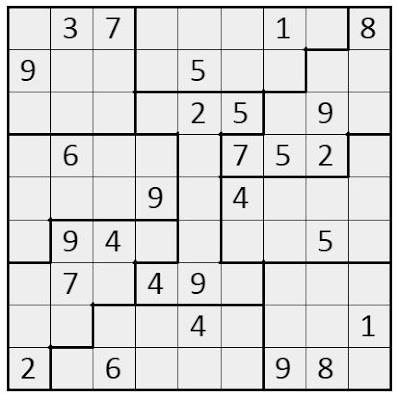### Sketching Jigsaw Sudoku - Using ProcessingJS / Part 2

It's almost a month since I last posted on Sketching Sudoku using ProcessingJS. Let's take that code ahead to draw a Jigsaw Sudoku.

Jigsaw Sudoku, also referred as Irregular Sudoku by some sites, also has 9x9 grid similar to a Classic Sudoku. What makes it different is the shape of 9 regions which were 3x3 grids in a classic one. In Jigsaw Sudoku each region would have 9 cells but grouped in somewhat random shapes.

Technically speaking, we had hard-coded the locations where the thick lines would be drawn in previous post. Now we would have to remove that and write a code that can draw a Jigsaw Sudoku. Let's target the Jigsaw Sudoku that appeared on sudoku.org.uk today.

Let's assign a number 1-9 to each region and represent the entire puzzle layout in a single string.
```
layout="111222223111222233111444333555543336555546666577746666777444888779999888799999888"
```

The command below will break the string into a two dimensional array of size 9x9. Of course we could have directly hand-coded the array instead of first writing it as a string, but imagine yourself doing that daily to encode the puzzles.

```layarr=layout.match(/.{1,9}/g).map(ret => ret.split(""));
```Now, while coding the Sudoku in previous post, we drew thick vertical lines on extreme left and then after every third cell. Similarly thick horizontal lines were drawn on the top and then after every three rows. This time we would follow a simple logic of drawing a thick line whenever there is a change of region in horizontal or vertical direction (the reason why we prepared the layout array)

So,the lines of code,in previous post, where we modified the stroke weights will look like:

```if (ctr2 == 0 || ctr2 == 9 || (ctr1 != 9 && layarr[ctr1][ctr2] != layarr[ctr1][ctr2-1])) { strokeWeight(3); }
.
.
if (ctr1 == 0 || ctr1 == 9 || layarr[ctr1][ctr2] != layarr[ctr1-1][ctr2]) { strokeWeight(3); }
.
.

```
Since this puzzle has a different set of "given" numbers let's also update the 'txt' variable as below, at relevant line.

```txt=" 37   1 89   5        25 9  6   752    9 4    94    5  7 49        4   12 6   98 "
```

... and voila, we have the Jigsaw Sudoku Puzzle drawnTo get the classic puzzle from last post all need to do is to encode the layout differently.

```txt = "  7  1 2 5   2 916     5 38 6   8  7   3 7   1  6   4 48 5     926 7   1 5 2  3  "
layout="111222333111222333111222333444555666444555666444555666777888999777888999777888999"
```

Entire draw() function reproduced below for clarity.

```  void draw() {
x = (width - 3) / 9;
txt=" 37   1 89   5        25 9  6   752    9 4    94    5  7 49        4   12 6   98 ";
layout="111222223111222233111444333555543336555546666577746666777444888779999888799999888";
layarr=layout.match(/.{1,9}/g).map(ret => ret.split(""));
a1 = txt.split("");
for (ctr1 = 0; ctr1 <= 9; ctr1++) {
for (ctr2 = 0; ctr2 <= 9; ctr2++) {
strokeWeight(1);
if (ctr2 == 0 || ctr2 == 9 || (ctr1 != 9 && layarr[ctr1][ctr2] != layarr[ctr1][ctr2-1])) { strokeWeight(3); }
line(ctr2 * x + 1, ctr1 * x, ctr2 * x + 1, (ctr1 + 1) * x);
strokeWeight(1);
if (ctr1 == 0 || ctr1 == 9 || layarr[ctr1][ctr2] != layarr[ctr1-1][ctr2]) { strokeWeight(3); }
line(ctr2 * x, ctr1 * x + 1, (ctr2 + 1) * x, ctr1 * x + 1);
if (ctr2 != 9 && ctr1 != 9) {
text(a1[ctr1 * 9 + ctr2], ctr2 * x + x / 2, ctr1 * x + x / 2);
}
}
}
}
```

Now What next? The Killer Sudoku in the below video, perhaps!